Last modified on 5 July 2018, at 03:41

# Dot productThis article/section deals with mathematical concepts appropriate for late high school or early college.

In the-dimensional Euclidean vector space, the dot product is defined for two vectorsandas follows:Unlike the cross product, the dot product is a scalar, not a vector, and has no direction. Also, unlike the cross product, the dot product is commutative.

For example, in 3-dimensional Euclidean space, let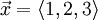and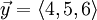. Then we can calculate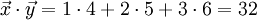.

The dot product of any vector with itself is the square of its norm.

In 2- and 3- dimensional Euclidean space, the relation between the dot product of two vectors and the angle between them is given by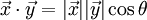where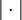is the vector norm and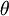is the angle between the vectors. Note that the vectors are perpendicular (or orthogonal) if and only if their dot product is zero.

This relationship can be extended to define the concept of the angle between vectors in higher-dimensional Euclidean vector spaces. Two vectors inare defined to be orthogonal if their dot product is zero.

The dot product is the best-known example of an inner product.

## Application

The dot product is useful in projecting one vector onto another, as in calculating the work done by applying a force to a particle. If you know the dot product of two vectors, then you can easily calculate the angle between the vectors.

The dot product can also be used to find other values through application of the Stokes' Theorem and other theorems.